# Plot of a scalar field [Graphical outputs.]

() More...

## Functions

void des_profile (const Scalar &uu, double r_min, double r_max, double theta, double phi, const char *nomy=0x0, const char *title=0x0, bool draw_bound=true)
Draws the profile of a Scalar along some radial axis determined by a fixed value of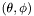(version with x-axis labelled with Lorene's unit of length).
void des_profile (const Scalar &uu, double r_min, double r_max, double scale, double theta, double phi, const char *nomx=0x0, const char *nomy=0x0, const char *title=0x0, bool draw_bound=true)
Draws the profile of a Scalar along some radial axis determined by a fixed value of(general version).
void des_profile_mult (const Scalar **uu, int nprof, double r_min, double r_max, const double *theta, const double *phi, double radial_scale=1, bool closeit=true, const char *nomy=0x0, const char *title=0x0, int ngraph=0, const char *nomx=0x0, const int *line_style=0x0, const char *device=0x0, bool draw_bound=true)
Draws the profile of Scalar 's along some radial axis determined by a fixed value of.
void des_points (const Scalar &uu, double theta=0, double phi=0, const char *nomy=0x0, const char *title=0x0, bool draw_bound=true)
Draws the grid points of a Scalar along some radial axis determined by a fixed value of(version with x-axis labelled with Lorene's unit of length).
void des_points (const Scalar &uu, double scale, double theta=0, double phi=0, const char *nomx=0x0, const char *nomy=0x0, const char *title=0x0, bool draw_bound=true)
Draws the grid points of a Scalar along some radial axis determined by a fixed value of(general version).
void save_profile (const Scalar &uu, double r_min, double r_max, double theta, double phi, const char *filename)
Saves in a file the profile of a Scalar along some radial axis determined by a fixed value of.
void des_meridian (const Scalar &uu, double r_min, double r_max, const char *nomy, int ngraph, const char *device=0x0, bool closeit=false, bool draw_bound=true)
Draws 5 profiles of a scalar field along various radial axes in two meridional planes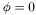and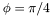.
void des_surface_x (const Scalar &defsurf, double x0, const char *device=0x0, int newgraph=3, double y_min=-1, double y_max=1, double z_min=-1, double z_max=1, const char *nomy=0x0, const char *nomz=0x0, const char *title=0x0, int nxpage=1, int nypage=1)
Basic routine for drawing a stellar surface in a plane X=constant.
void des_surface_y (const Scalar &defsurf, double y0, const char *device=0x0, int newgraph=3, double x_min=-1, double x_max=1, double z_min=-1, double z_max=1, const char *nomx=0x0, const char *nomz=0x0, const char *title=0x0, int nxpage=1, int nypage=1)
Basic routine for drawing a stellar surface in a plane Y=constant.
void des_surface_z (const Scalar &defsurf, double z0, const char *device=0x0, int newgraph=3, double x_min=-1, double x_max=1, double y_min=-1, double y_max=1, const char *nomx=0x0, const char *nomz=0x0, const char *title=0x0, int nxpage=1, int nypage=1)
Basic routine for drawing a stellar surface in a plane Z=constant.
void des_coupe_x (const Scalar &uu, double x0, int nzdes, const char *title=0x0, const Scalar *defsurf=0x0, double zoom=1.2, bool draw_bound=true, int ncour=15, int ny=100, int nz=100)
Draws isocontour lines of a Scalar in a plane X=constant.
void des_coupe_x (const Scalar &uu, double x0, double y_min, double y_max, double z_min, double z_max, const char *title=0x0, const Scalar *defsurf=0x0, bool draw_bound=true, int ncour=15, int ny=100, int nz=100)
Draws isocontour lines of a Scalar in a plane X=constant within a specified graphic window.
void des_coupe_y (const Scalar &uu, double y0, int nzdes, const char *title=0x0, const Scalar *defsurf=0x0, double zoom=1.2, bool draw_bound=true, int ncour=15, int nx=100, int nz=100)
Draws isocontour lines of a Scalar in a plane Y=constant.
void des_coupe_y (const Scalar &uu, double y0, double x_min, double x_max, double z_min, double z_max, const char *title=0x0, const Scalar *defsurf=0x0, bool draw_bound=true, int ncour=15, int nx=100, int nz=100)
Draws isocontour lines of a Scalar in a plane Y=constant within a specified graphic window.
void des_coupe_z (const Scalar &uu, double z0, int nzdes, const char *title=0x0, const Scalar *defsurf=0x0, double zoom=1.2, bool draw_bound=true, int ncour=15, int nx=100, int ny=100)
Draws isocontour lines of a Scalar in a plane Z=constant.
void des_coupe_z (const Scalar &uu, double z0, double x_min, double x_max, double y_min, double y_max, const char *title=0x0, const Scalar *defsurf=0x0, bool draw_bound=true, int ncour=15, int nx=100, int ny=100)
Draws isocontour lines of a Scalar in a plane Z=constant within a specified graphic window.

()

## Function Documentation

 void des_coupe_x ( const Scalar & uu, double x0, double y_min, double y_max, double z_min, double z_max, const char * title = 0x0, const Scalar * defsurf = 0x0, bool draw_bound = true, int ncour = 15, int ny = 100, int nz = 100 )

Draws isocontour lines of a Scalar in a plane X=constant within a specified graphic window.

X is the Cartesian coordinate relative to the absolute frame. Solid (resp. dashed) lines correspond to positive (resp. negative) values of the field.

Parameters:
 uu [input] Scalar to be drawn x0 [input] value of the coordinate X which defines the plane of the drawing y_min [input] lowest value of absol. coord. Y y_max [input] highest value of absol. coord. Y z_min [input] lowest value of absol. coord. Z z_max [input] highest value of absol. coord. Z title [input] title of the figure (default value = 0x0, corresponds to no title) defsurf [input] pointer on a Scalar giving the definition of the surface: the surface is defined as the location where this Scalar vanishes (default value = 0x0, corresponds to no surface plot) draw_bound [input] true for drawing the boundaries of the various domains (default value = true) ncour [input] number of isocontour lines (default value = 15) ny [input] number of points in the Y direction (default value = 100) nz [input] number of points in the Z direction (default value = 100)
 void des_coupe_x ( const Scalar & uu, double x0, int nzdes, const char * title = 0x0, const Scalar * defsurf = 0x0, double zoom = 1.2, bool draw_bound = true, int ncour = 15, int ny = 100, int nz = 100 )

Draws isocontour lines of a Scalar in a plane X=constant.

X is the Cartesian coordinate relative to the absolute frame. Solid (resp. dashed) lines correspond to positive (resp. negative) values of the field.

Parameters:
 uu [input] Scalar to be drawn x0 [input] value of the coordinate X which defines the plane of the drawing nzdes [input] number of domains for which the plot is performed: the size of the graphic window is determined so that the nzdes innermost domains fit in it (for zoom = 1.) title [input] title of the figure (default value = 0x0, corresponds to no title) defsurf [input] pointer on a Scalar giving the definition of the surface: the surface is defined as the location where this Scalar vanishes (default value = 0x0, corresponds to no surface plot) zoom [input] Factor by which the size of the graphic window (determined from the size of the nzdes innermost domains) is multiplied (default value = 1.2) draw_bound [input] true for drawing the boundaries of the various domains (default value = true) ncour [input] number of isocontour lines (default value = 15) ny [input] number of points in the Y direction (default value = 100) nz [input] number of points in the Z direction (default value = 100)
 void des_coupe_y ( const Scalar & uu, double y0, double x_min, double x_max, double z_min, double z_max, const char * title = 0x0, const Scalar * defsurf = 0x0, bool draw_bound = true, int ncour = 15, int nx = 100, int nz = 100 )

Draws isocontour lines of a Scalar in a plane Y=constant within a specified graphic window.

Y is the Cartesian coordinate relative to the absolute frame. Solid (resp. dashed) lines correspond to positive (resp. negative) values of the field.

Parameters:
 uu [input] Scalar to be drawn y0 [input] value of the coordinate Y which defines the plane of the drawing x_min [input] lowest value of absol. coord. X x_max [input] highest value of absol. coord. X z_min [input] lowest value of absol. coord. Z z_max [input] highest value of absol. coord. Z title [input] title of the figure (default value = 0x0, corresponds to no title) defsurf [input] pointer on a Scalar giving the definition of the surface: the surface is defined as the location where this Scalar vanishes (default value = 0x0, corresponds to no surface plot) draw_bound [input] true for drawing the boundaries of the various domains (default value = true) ncour [input] number of isocontour lines (default value = 15) nx [input] number of points in the X direction (default value = 100) nz [input] number of points in the Z direction (default value = 100)
 void des_coupe_y ( const Scalar & uu, double y0, int nzdes, const char * title = 0x0, const Scalar * defsurf = 0x0, double zoom = 1.2, bool draw_bound = true, int ncour = 15, int nx = 100, int nz = 100 )

Draws isocontour lines of a Scalar in a plane Y=constant.

Y is the Cartesian coordinate relative to the absolute frame. Solid (resp. dashed) lines correspond to positive (resp. negative) values of the field.

Parameters:
 uu [input] Scalar to be drawn y0 [input] value of the coordinate Y which defines the plane of the drawing nzdes [input] number of domains for which the plot is performed: the size of the graphic window is determined so that the nzdes innermost domains fit in it (for zoom = 1.) title [input] title of the figure (default value = 0x0, corresponds to no title) defsurf [input] pointer on a Scalar giving the definition of the surface: the surface is defined as the location where this Scalar vanishes (default value = 0x0, corresponds to no surface plot) zoom [input] Factor by which the size of the graphic window (determined from the size of the nzdes innermost domains) is multiplied (default value = 1.2) draw_bound [input] true for drawing the boundaries of the various domains (default value = true) ncour [input] number of isocontour lines (default value = 15) nx [input] number of points in the X direction (default value = 100) nz [input] number of points in the Z direction (default value = 100)
 void des_coupe_z ( const Scalar & uu, double z0, double x_min, double x_max, double y_min, double y_max, const char * title = 0x0, const Scalar * defsurf = 0x0, bool draw_bound = true, int ncour = 15, int nx = 100, int ny = 100 )

Draws isocontour lines of a Scalar in a plane Z=constant within a specified graphic window.

Z is the Cartesian coordinate relative to the absolute frame. Solid (resp. dashed) lines correspond to positive (resp. negative) values of the field.

Parameters:
 uu [input] Scalar to be drawn z0 [input] value of the coordinate Z which defines the plane of the drawing x_min [input] lowest value of absol. coord. X x_max [input] highest value of absol. coord. X y_min [input] lowest value of absol. coord. Y y_max [input] highest value of absol. coord. Y title [input] title of the figure (default value = 0x0, corresponds to no title) defsurf [input] pointer on a Scalar giving the definition of the surface: the surface is defined as the location where this Scalar vanishes (default value = 0x0, corresponds to no surface plot) draw_bound [input] true for drawing the boundaries of the various domains (default value = true) ncour [input] number of isocontour lines (default value = 15) nx [input] number of points in the X direction (default value = 100) ny [input] number of points in the Y direction (default value = 100)
 void des_coupe_z ( const Scalar & uu, double z0, int nzdes, const char * title = 0x0, const Scalar * defsurf = 0x0, double zoom = 1.2, bool draw_bound = true, int ncour = 15, int nx = 100, int ny = 100 )

Draws isocontour lines of a Scalar in a plane Z=constant.

Z is the Cartesian coordinate relative to the absolute frame. Solid (resp. dashed) lines correspond to positive (resp. negative) values of the field.

Parameters:
 uu [input] Scalar to be drawn z0 [input] value of the coordinate Z which defines the plane of the drawing nzdes [input] number of domains for which the plot is performed: the size of the graphic window is determined so that the nzdes innermost domains fit in it (for zoom = 1.) title [input] title of the figure (default value = 0x0, corresponds to no title) defsurf [input] pointer on a Scalar giving the definition of the surface: the surface is defined as the location where this Scalar vanishes (default value = 0x0, corresponds to no surface plot) zoom [input] Factor by which the size of the graphic window (determined from the size of the nzdes innermost domains) is multiplied (default value = 1.2) draw_bound [input] true for drawing the boundaries of the various domains (default value = true) ncour [input] number of isocontour lines (default value = 15) nx [input] number of points in the X direction (default value = 100) ny [input] number of points in the Y direction (default value = 100)
 void des_meridian ( const Scalar & uu, double r_min, double r_max, const char * nomy, int ngraph, const char * device = 0x0, bool closeit = false, bool draw_bound = true )

Draws 5 profiles of a scalar field along various radial axes in two meridional planesand.

For, 3 profiles are drawn, corresponding to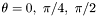, whereas for, 2 profiles are drawn, corresponding to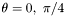.

Parameters:
 uu [input] Scalar field to be drawn r_min [input] Minimal value of r for the drawing r_max [input] Maximal value of r for the drawing nomy [input] y legend of the figure (default value = 0x0, corresponds to no y legend) ngraph [input] Index of the graphic device (in the range [0,99]) to be used for the plot: if this device has never been used or is closed, it will be opened. device [input] type of PGPLOT device: 0x0 (default value) will result in interactive choice; "/xwin" in X-Window display; "filename.eps/cps" in Encapsulated PostScript output and "/n" in no output. closeit [input] determines whether the graphic device must be closed or not after the plot has been performed draw_bound [input] true for drawing the boundaries of the various domains (default value = true)
 void des_points ( const Scalar & uu, double scale, double theta = 0, double phi = 0, const char * nomx = 0x0, const char * nomy = 0x0, const char * title = 0x0, bool draw_bound = true )

Draws the grid points of a Scalar along some radial axis determined by a fixed value of(general version).

Parameters:
 uu [input] Scalar to be drawn scale scale factor for the radius in the plot theta [input] Value of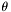which defines the profile axis phi [input] Value of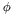which defines the profile axis nomx [input] x legend of the figure (default value = 0x0, corresponds to no x legend) nomy [input] y legend of the figure (default value = 0x0, corresponds to no y legend) title [input] title of the figure (default value = 0x0, corresponds to no title) draw_bound [input] true for drawing the boundaries of the various domains (default value = true)
 void des_points ( const Scalar & uu, double theta = 0, double phi = 0, const char * nomy = 0x0, const char * title = 0x0, bool draw_bound = true )

Draws the grid points of a Scalar along some radial axis determined by a fixed value of(version with x-axis labelled with Lorene's unit of length).

Parameters:
 uu [input] Scalar to be drawn theta [input] Value ofwhich defines the profile axis phi [input] Value ofwhich defines the profile axis nomy [input] y legend of the figure (default value = 0x0, corresponds to no y legend) title [input] title of the figure (default value = 0x0, corresponds to no title) draw_bound [input] true for drawing the boundaries of the various domains (default value = true)
 void des_profile ( const Scalar & uu, double r_min, double r_max, double scale, double theta, double phi, const char * nomx = 0x0, const char * nomy = 0x0, const char * title = 0x0, bool draw_bound = true )

Draws the profile of a Scalar along some radial axis determined by a fixed value of(general version).

Parameters:
 uu [input] Scalar to be drawn r_min [input] Minimal value of r for the drawing r_max [input] Maximal value of r for the drawing scale scale factor for the radius in the plot theta [input] Value ofwhich defines the profile axis phi [input] Value ofwhich defines the profile axis nomx [input] x legend of the figure (default value = 0x0, corresponds to no x legend) nomy [input] y legend of the figure (default value = 0x0, corresponds to no y legend) title [input] title of the figure (default value = 0x0, corresponds to no title) draw_bound [input] true for drawing the boundaries of the various domains (default value = true)
 void des_profile ( const Scalar & uu, double r_min, double r_max, double theta, double phi, const char * nomy = 0x0, const char * title = 0x0, bool draw_bound = true )

Draws the profile of a Scalar along some radial axis determined by a fixed value of(version with x-axis labelled with Lorene's unit of length).

Parameters:
 uu [input] Scalar to be drawn r_min [input] Minimal value of r for the drawing r_max [input] Maximal value of r for the drawing theta [input] Value ofwhich defines the profile axis phi [input] Value ofwhich defines the profile axis nomy [input] y legend of the figure (default value = 0x0, corresponds to no y legend) title [input] title of the figure (default value = 0x0, corresponds to no title) draw_bound [input] true for drawing the boundaries of the various domains (default value = true)
 void des_profile_mult ( const Scalar ** uu, int nprof, double r_min, double r_max, const double * theta, const double * phi, double radial_scale = 1, bool closeit = true, const char * nomy = 0x0, const char * title = 0x0, int ngraph = 0, const char * nomx = 0x0, const int * line_style = 0x0, const char * device = 0x0, bool draw_bound = true )

Draws the profile of Scalar 's along some radial axis determined by a fixed value of.

Parameters:
 uu [input] Array (size nprof ) containing the addresses of the Scalar to be drawn nprof [input] Number of Scalar 's to be drawn r_min [input] Minimal value of r for the drawing r_max [input] Maximal value of r for the drawing theta [input] Array (size nprof ) of the values ofdefining the profile axis for each plot: the line no.i represents the scalar uu[i] along the direction (theta[i],phi[i]) phi [input] Array (size nprof ) of the values ofdefining the profile axis for each plot: the line no.i represents the scalar uu[i] along the direction (theta[i],phi[i]) radial_scale [input] factor by which the values of r are to be multiplied to get the abscidia scale closeit [input] determines whether the graphic device must be closed or not after the plot has been performed nomy [input] y legend of the figure (default value = 0x0, corresponds to no y legend) title [input] title of the figure (default value = 0x0, corresponds to no title) ngraph [input] Index of the graphic device (in the range [0,99]) to be used for the plot: if this device has never been used or is closed, it will be opened. nomx [input] x legend of the figure (default value = 0x0, corresponds to "r") line_style [input] Array (size nprof ) defining the line style for each plot: the possible values are line_style[i] = 1 (full line), 2 (dashed), 3 (dot-dash-dot-dash), 4 (dotted), 5 (dash-dot-dot-dot). The default value = 0x0 corresponds to a cyclic sequence of the above styles. device [input] type of PGPLOT device: 0x0 (default value) will result in interactive choice; "/xwin" in X-Window display; "filename.eps/cps" in Encapsulated PostScript output and "/n" in no output. draw_bound [input] true for drawing the boundaries of the various domains (default value = true)
 void des_surface_x ( const Scalar & defsurf, double x0, const char * device = 0x0, int newgraph = 3, double y_min = -1, double y_max = 1, double z_min = -1, double z_max = 1, const char * nomy = 0x0, const char * nomz = 0x0, const char * title = 0x0, int nxpage = 1, int nypage = 1 )

Basic routine for drawing a stellar surface in a plane X=constant.

X is the Cartesian coordinate relative to the absolute frame. The surface to be drawn is defined as the location where a given scalar field vanishes.

Parameters:
 defsurf [input] field defining the surface: the surface is defined as the location where this Scalar vanishes x0 [input] value of the absolute coordinate X which defines the plane of the drawing device [input] PGPLOT device (default value = 0x0) newgraph [input] controls the opening/closing of the graphic device: 0 : does nothing (the device must be already opened) 1 : opens the device but does not close it at the end 2 : closes the device at the end but does not open it at the beginning 3 (default value) : opens and closes the device y_min [input] lowest value of absol. coord. Y (default value = -1) y_max [input] highest value of absol. coord. Y (default value = 1) z_min [input] lowest value of absol. coord. Z (default value = -1) z_max [input] highest value of absol. coord. Z (default value = 1) nomy [input] y legend of the figure (default value = 0x0) nomz [input] z legend of the figure (default value = 0x0) title [input] title of the figure (default value = 0x0) nxpage [input] number of graphs in the horizontal direction of the display window (meaningfull only if newgraph = 1 or 3) (default value = 1) nypage [input] number of graphs in the vertical direction of the display window (meaningfull only if newgraph = 1 or 3) (default value = 1)
 void des_surface_y ( const Scalar & defsurf, double y0, const char * device = 0x0, int newgraph = 3, double x_min = -1, double x_max = 1, double z_min = -1, double z_max = 1, const char * nomx = 0x0, const char * nomz = 0x0, const char * title = 0x0, int nxpage = 1, int nypage = 1 )

Basic routine for drawing a stellar surface in a plane Y=constant.

Y is the Cartesian coordinate relative to the absolute frame. The surface to be drawn is defined as the location where a given scalar field vanishes.

Parameters:
 defsurf [input] field defining the surface: the surface is defined as the location where this Scalar vanishes y0 [input] value of the coordinate Y which defines the plane of the drawing device [input] PGPLOT device (default value = 0x0) newgraph [input] controls the opening/closing of the graphic device: 0 : does nothing (the device must be already opened) 1 : opens the device but does not close it at the end 2 : closes the device at the end but does not open it at the beginning 3 (default value) : opens and closes the device x_min [input] lowest value of absol. coord. X (default value = -1) x_max [input] highest value of absol. coord. X (default value = 1) z_min [input] lowest value of absol. coord. Z (default value = -1) z_max [input] highest value of absol. coord. Z (default value = 1) nomx [input] x legend of the figure (default value = 0x0) nomz [input] z legend of the figure (default value = 0x0) title [input] title of the figure (default value = 0x0) nxpage [input] number of graphs in the horizontal direction of the display window (meaningfull only if newgraph = 1 or 3) (default value = 1) nypage [input] number of graphs in the vertical direction of the display window (meaningfull only if newgraph = 1 or 3) (default value = 1)
 void des_surface_z ( const Scalar & defsurf, double z0, const char * device = 0x0, int newgraph = 3, double x_min = -1, double x_max = 1, double y_min = -1, double y_max = 1, const char * nomx = 0x0, const char * nomz = 0x0, const char * title = 0x0, int nxpage = 1, int nypage = 1 )

Basic routine for drawing a stellar surface in a plane Z=constant.

Z is the Cartesian coordinate relative to the absolute frame. The surface to be drawn is defined as the location where a given scalar field vanishes.

Parameters:
 defsurf [input] field defining the surface: the surface is defined as the location where this Scalar vanishes z0 [input] value of the coordinate Z which defines the plane of the drawing device [input] PGPLOT device (default value = 0x0) newgraph [input] controls the opening/closing of the graphic device: 0 : does nothing (the device must be already opened) 1 : opens the device but does not close it at the end 2 : closes the device at the end but does not open it at the beginning 3 (default value) : opens and closes the device x_min [input] lowest value of absol. coord. X (default value = -1) x_max [input] highest value of absol. coord. X (default value = 1) y_min [input] lowest value of absol. coord. Y (default value = -1) y_max [input] highest value of absol. coord. Y (default value = 1) nomx [input] x legend of the figure (default value = 0x0) nomy [input] y legend of the figure (default value = 0x0) title [input] title of the figure (default value = 0x0) nxpage [input] number of graphs in the horizontal direction of the display window (meaningfull only if newgraph = 1 or 3) (default value = 1) nypage [input] number of graphs in the vertical direction of the display window (meaningfull only if newgraph = 1 or 3) (default value = 1)
 void save_profile ( const Scalar & uu, double r_min, double r_max, double theta, double phi, const char * filename )

Saves in a file the profile of a Scalar along some radial axis determined by a fixed value of.

Parameters:
 uu [input] Scalar to be drawn r_min [input] Minimal value of r r_max [input] Maximal value of r theta [input] Value ofwhich defines the profile axis phi [input] Value ofwhich defines the profile axis filename [input] root of the filename

Generated on 7 Dec 2019 for LORENE by1.6.1